Publicité

# R programming Language , Rahul Singh

10 Mar 2016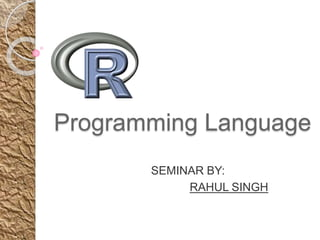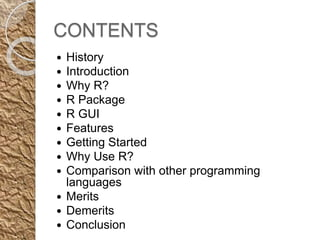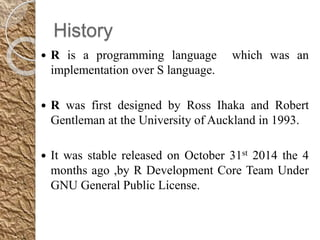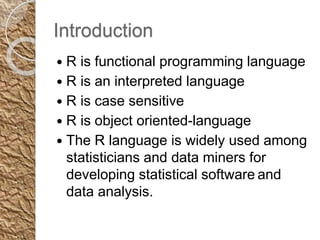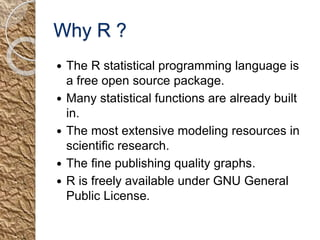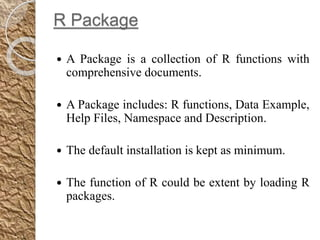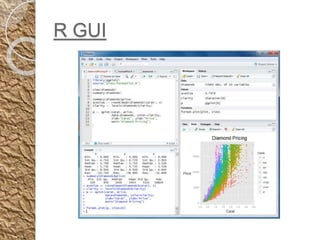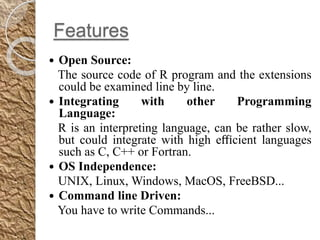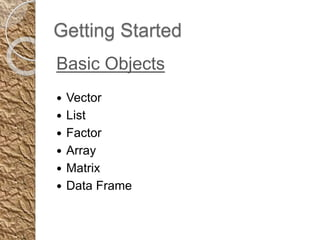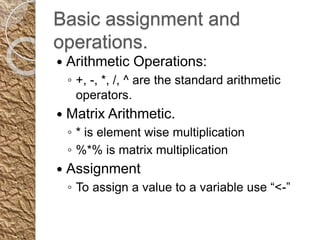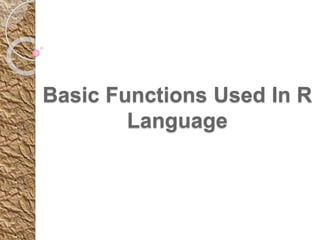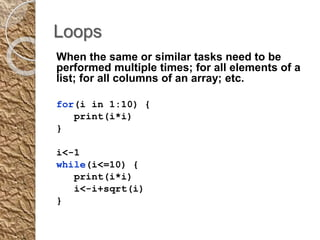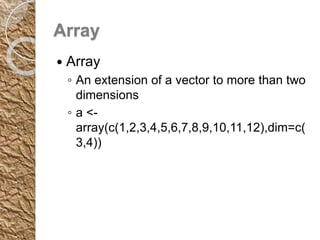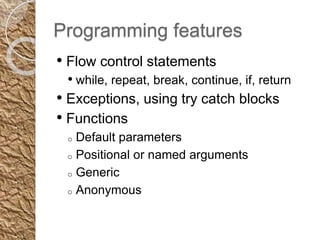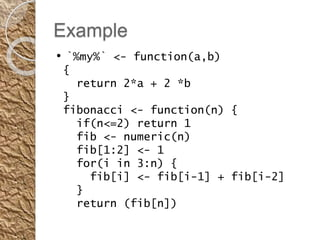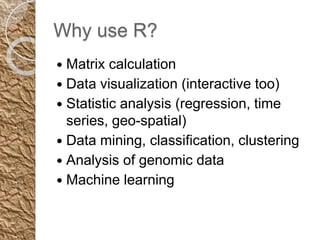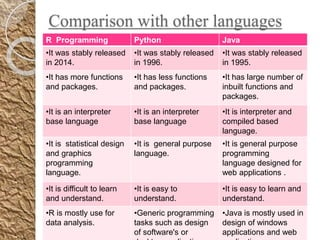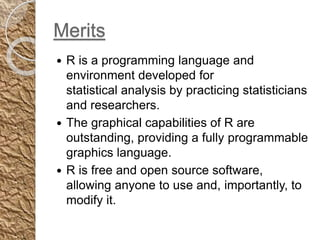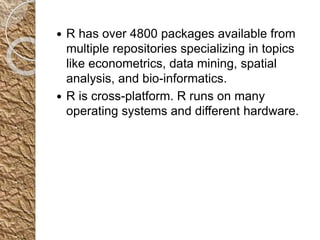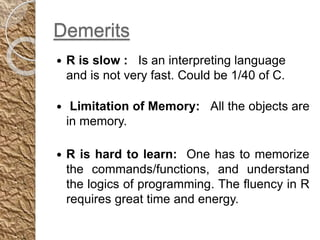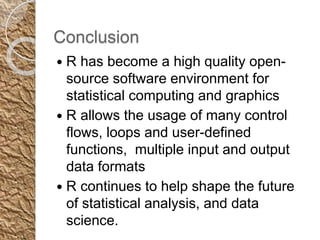1 sur 22
Publicité

### R programming Language , Rahul Singh

1. Programming Language SEMINAR BY: RAHUL SINGH
2. CONTENTS  History  Introduction  Why R?  R Package  R GUI  Features  Getting Started  Why Use R?  Comparison with other programming languages  Merits  Demerits  Conclusion
3. History  R is a programming language which was an implementation over S language.  R was first designed by Ross Ihaka and Robert Gentleman at the University of Auckland in 1993.  It was stable released on October 31st 2014 the 4 months ago ,by R Development Core Team Under GNU General Public License.
4. Introduction  R is functional programming language  R is an interpreted language  R is case sensitive  R is object oriented-language  The R language is widely used among statisticians and data miners for developing statistical software and data analysis.
5. Why R ?  The R statistical programming language is a free open source package.  Many statistical functions are already built in.  The most extensive modeling resources in scientiﬁc research.  The ﬁne publishing quality graphs.  R is freely available under GNU General Public License.
6. R Package  A Package is a collection of R functions with comprehensive documents.  A Package includes: R functions, Data Example, Help Files, Namespace and Description.  The default installation is kept as minimum.  The function of R could be extent by loading R packages.
7. R GUI
8. Features  Open Source: The source code of R program and the extensions could be examined line by line.  Integrating with other Programming Language: R is an interpreting language, can be rather slow, but could integrate with high eﬃcient languages such as C, C++ or Fortran.  OS Independence: UNIX, Linux, Windows, MacOS, FreeBSD...  Command line Driven: You have to write Commands...
9. Getting Started Basic Objects  Vector  List  Factor  Array  Matrix  Data Frame
10. Basic assignment and operations.  Arithmetic Operations: ◦ +, -, *, /, ^ are the standard arithmetic operators.  Matrix Arithmetic. ◦ * is element wise multiplication ◦ %*% is matrix multiplication  Assignment ◦ To assign a value to a variable use “<-”
11. Basic Functions Used In R Language
12. Loops When the same or similar tasks need to be performed multiple times; for all elements of a list; for all columns of an array; etc. for(i in 1:10) { print(i*i) } i<-1 while(i<=10) { print(i*i) i<-i+sqrt(i) }
13. Array  Array ◦ An extension of a vector to more than two dimensions ◦ a <- array(c(1,2,3,4,5,6,7,8,9,10,11,12),dim=c( 3,4))
14. Programming features • Flow control statements • while, repeat, break, continue, if, return • Exceptions, using try catch blocks • Functions o Default parameters o Positional or named arguments o Generic o Anonymous
15. Example • `%my%` <- function(a,b) { return 2*a + 2 *b } fibonacci <- function(n) { if(n<=2) return 1 fib <- numeric(n) fib[1:2] <- 1 for(i in 3:n) { fib[i] <- fib[i-1] + fib[i-2] } return (fib[n])
16. Why use R?  Matrix calculation  Data visualization (interactive too)  Statistic analysis (regression, time series, geo-spatial)  Data mining, classification, clustering  Analysis of genomic data  Machine learning
17. Comparison with other languages R Programming Python Java •It was stably released in 2014. •It was stably released in 1996. •It was stably released in 1995. •It has more functions and packages. •It has less functions and packages. •It has large number of inbuilt functions and packages. •It is an interpreter base language •It is an interpreter base language •It is interpreter and compiled based language. •It is statistical design and graphics programming language. •It is general purpose language. •It is general purpose programming language designed for web applications . •It is difficult to learn and understand. •It is easy to understand. •It is easy to learn and understand. •R is mostly use for data analysis. •Generic programming tasks such as design of software's or •Java is mostly used in design of windows applications and web
18. Merits  R is a programming language and environment developed for statistical analysis by practicing statisticians and researchers.  The graphical capabilities of R are outstanding, providing a fully programmable graphics language.  R is free and open source software, allowing anyone to use and, importantly, to modify it.
19.  R has over 4800 packages available from multiple repositories specializing in topics like econometrics, data mining, spatial analysis, and bio-informatics.  R is cross-platform. R runs on many operating systems and different hardware.
20. Demerits  R is slow : Is an interpreting language and is not very fast. Could be 1/40 of C.  Limitation of Memory: All the objects are in memory.  R is hard to learn: One has to memorize the commands/functions, and understand the logics of programming. The ﬂuency in R requires great time and energy.
21. Conclusion  R has become a high quality open- source software environment for statistical computing and graphics  R allows the usage of many control flows, loops and user-defined functions, multiple input and output data formats  R continues to help shape the future of statistical analysis, and data science.
22. Thanks..
Publicité